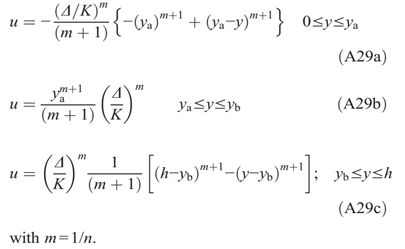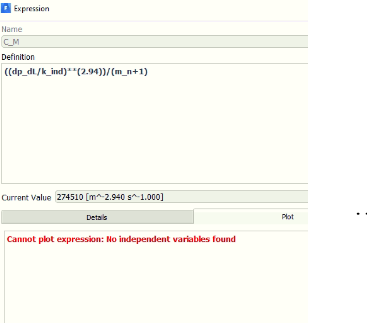## Fluids

•ecrod
Subscriber

I am trying to use a named expression as velocity boundary condition at inlet. My expression is working fine as you can see in the plot.

•YasserSelima
Subscriber
How is u_a, u_b and u_p defined?n
•YasserSelima
Subscriber
by looking back at the photos you provided, it is a matter of defining real and integer.nnGo to u_a definition and make the units [m/s] ... do not have 1.000. Same for u_b and u_pn
•ecrod
SubscriberAbove to below are u_a, u_p and u_b. All of them have the same multiplier parameter as function of pressure gradient ((DELTA/K)^m)/(m+1). I beleive that K [Pa.s^0.34] can be a cause to velocity as [m s^-1.000]. Here are the units of this term ((DELTA/K)^m)/(m+1).nnn
•YasserSelima
Subscriber
try thisnu_vel * 1 [m/s] / 1 [m s^-1.000]nlet me know if it worksn
•ecrod
Subscriber
Hello Yasser,nIt does not work. This problem is due to Herschel-Bulkley consistency index definition K [Pa*s^n] in named expressions, because the flow index n = 0.34 is real number.nProbably it is not possible to use the above velocity equations using Named Expressions, maybe only UDF. However, I do not know anything about UDF.nThanks.nn
•ecrod
Subscriber
The solution was to use everything dimensionless, included Y coordinate. In the end I used only u_vel [m s^-1] unit.nThanks your help Yasser.n
•YasserSelima
Subscriber
You are welcome. I was actually into suggesting this but I thought it is less hassle to try changing the units first n
•DrAmine
Ansys Employee
Thanks for getting back with your solution. Yes I recommend to keep things dimensionsless when running into troubles or to correct at least the output by just adjusting the units as I think checking the whole parts of the formula might be complicated.n